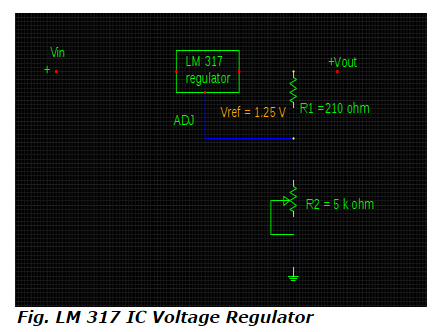# Numerical - maximum and minimum output voltages for IC voltage regulator with adjustable current and input voltage

What is the maximum and minimum output voltages for IC voltage regulator shown below if adjustable current and input voltage are 98.5 µA and 40 V respectively with the variable range of resistances from zero to 5 k ohm ?Options:

a. 30.16 V & 1.25
b. 29.66 V & 0 V
c. 27.99 V & 0 V
d. 31.49 V & 1.25V

Correct Answer: d. 31.49 V & 1.25V

Explanation

According to the equation of output voltage for IC voltage regulator,

Vout = Vref [ 1+ (R2/ R1) ] + IADJ. R2

Considering R2 at its minimum value = 0 ohm, the output voltage is evaluated by,

Vout (min) = 1.25 [ 1 + (0 / 210 )] + (98.5 x10-6 ) x 0
= 1.25 V

For maximum value of R2 = 5 k ohm, the output voltage is,

Vout (max) = 1.25 [ 1 + (5 x 103 / 210)] + (98.5 x 10-6 ) x 5 x 103

= 1.25 [ 1+ (5000 / 210) ] + (98.5 x 10-6 ) x 5000
= 31 + 0.4925
= 31. 49 V